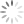Loading, please wait...## Operators

Introduction To Operators In SQL

Introduction:
In this article, you will learn, what are SQL Operators and different types of operators.

An operator is used for performing the certain operation on one or more operands and then return a result. The operators are divided and indexed, according to primary order. The operators are divided into eight different parts: assignment, arithmetic, relational, logical, bitwise, compound assignment, conditional and type comparison operators.

These operators are used to find the terms in SQL statement and to handle as an agreement for many terms in a statement. We mainly use three operators, which are-

• Arithmetic operators
• Comparison operators
• Logical operators

Arithmetic Operators

Arithmetic operators perform only the mathematical operations.

• (+ -) : When we add some values, we use this operator.

Example: x + y= 20

• (- -) : When we subtract some values, we use this operator.

Example: x - y = 20

• (* -) : When we multiply some values, we use this operator.

Example: x * y= 20

• (/ -) : When we divide some values, we use this operator.

Example: x / y= 20

• (% -) : This operator is used to find the percentage of one or more operands.

Example: x % y= 20

Comparison Operators

This operator is used to perform only compare operation.

• (= -) : It compares the value of two operands, if the values are same for both the operands, it gives true.

Example: (10 = 10) is true.

• (!= -) : It compares the value of two operands. If the values are not same, it gives false.

Example: (x != y) is not true.

• (<> -) : It compares the two operands. If the values are not equal for both the operands, it gives true.

Example: ( x<> y) is true.

• (> -) : In the comparison of an operand, if the value of the first operand is larger than the second operand, it means our condition becomes true.

Example: (x > y) is not true.

• (< -) : If the value of the first operand is smaller than the second operand, it means the condition becomes true.

Example: (x < y) is true.

• (>= ) : In the comparison of operand, if the value of the first operand is large and equal to the second operand, it means our condition becomes true.

Example: (x >= y) is not true.

• (<= ) : In the comparison of an operand, if the value of the first operand is lower or equal to the second operand, it means our condition becomes true.

Example: (x <= y) is true.

• (!< ) : In the comparison of an operand, if the value of the first operand is not lower, the value of the second operand means our condition becomes true.

Example: (x !< y) is false.

• ( !> ) : When we check first and second operand, the second operand is large or not. If our answer is yes, the condition becomes true.

Example: (x !> y) is true.

Logical Operators:

This operator is used to perform only the Logical operation.

• ALL : In this operator, we compare single value from all the values in another column.

• AND : In this operator, use we include many operations in SQL statement with Where clause.

• ANY : This operator use to join the values with another suitable values.

• BETWEEN : This operator is used to search for values in the particular set. And it gives also lowest and largest value in the data.

• EXISTS : This operator is used to find a row in a particular table, according to our condition.

• IN : This operator is used to compare a value to a list of the natural values.

• LIKE : This operator is used to check the same value within the multiple tables.

• NOT : This operator is used to reverse the means of the logical operator.

• OR : This operator is used to join many terms in SQL statement.

• IS NULL : This operator is used to analyze a value with a NULL value.

• UNIQUE : This operator is used to find the row in the particular table.

Summary:
Thus, we learnt that an operator is used to perform many operations on SQL statements.